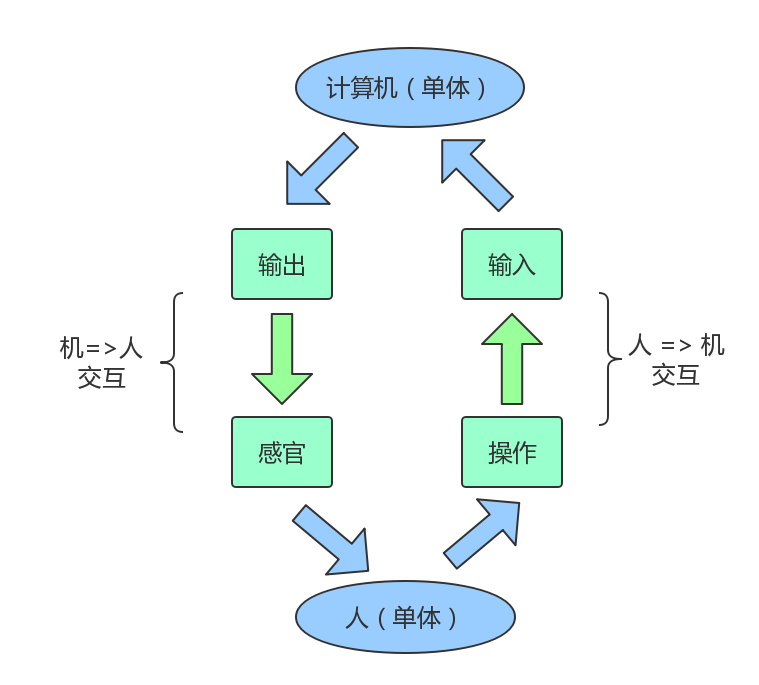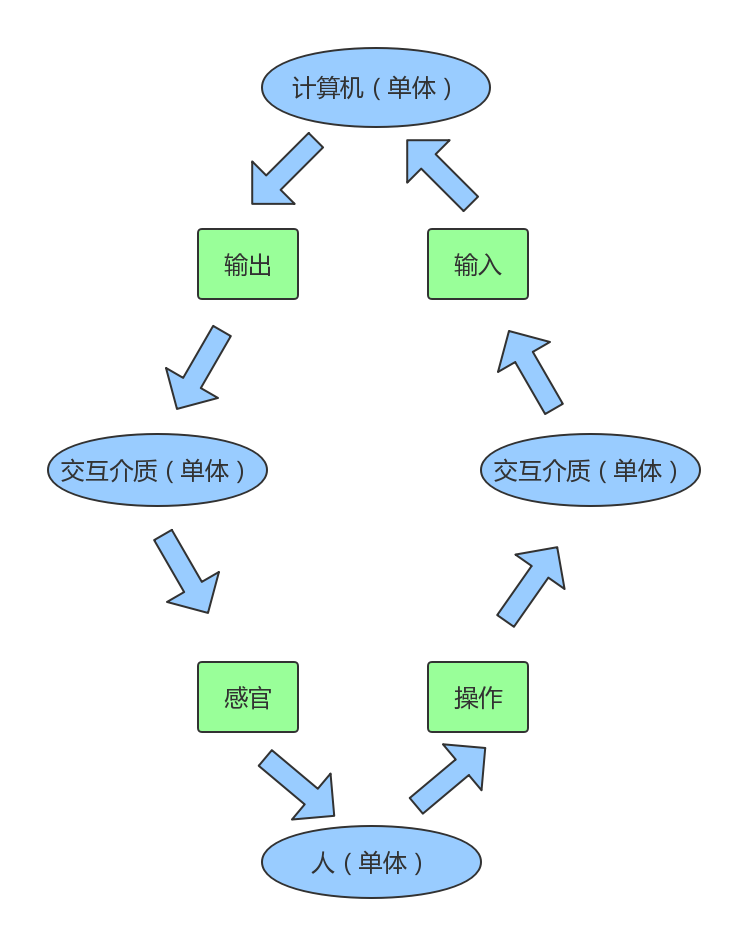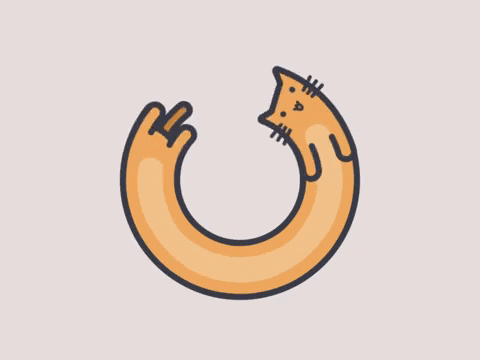# 单体模型

# 个体

function 人体(听觉, 视觉, 触觉, ...其他感觉) {
传递(听觉, 视觉, 触觉, ...其他感觉);
反馈 = 处理(听觉, 视觉, 触觉, ...其他感觉);
return 反馈;
}

function 个体(输入) {
输出 = 处理(输入);
return 输出;
}

# 群体

# 2. 微观角度

// 1. 假设个体间满足串行关系
function 群体(输入){
个体1输出 = 个体1处理(输入);
个体2输出 = 个体2处理(个体1输出);
...
个体n输出 = 个体n处理(个体n-1输出);
return 个体n输出;
}

// 2. 假设个体间满足并行关系
function 群体(输入){
// 拆分给各个个体的输入，分别处理
for(var i = 0; i < 个体.length; i++){
个体i输入 = 获取个体输入(输入, i);
个体i输出 = 个体i处理(个体i输入)
}
// 汇总输出
总输出 = 汇总([...各个个体的输出]);
return 总输出;
}

// 单体模型
function 单体(输入) {
输出 = 处理(输入);
return 输出;
}

# 交互

# 人机交互

• 人 => 机：通过操作对机器进行输入，例如从键盘输入内容、用鼠标点击某些元素等
• 机 => 人：机器拿到输入内容，根据程序进行计算，可通过显示器、声音设备进行输出function (输入) {
输出 = 处理(输入);
return 输出;
}

function (输入) {
输出 = 处理(输入);
return 输出;
}

// 1. 输入从机开始

// 2. 输入从人开始

# 多体交互

const 单体1输入 = 汇总(...其他n-1个单体的输出);

function 交互介质(单体输出) {
单体输入 = 处理(单体输出);
return 单体输入;
}# 流

function 监听器(输入流, 单体) {
输入流.变更 = 输入流 => {
单体(输入流);
};
}

function 添加流处理(...n个单体){
// 注册流的链用方法
n个单体.forEach((单体, n) => {.单体n = function() {
this = 单体(this);
return this;
}
})
}

...

# 光与电的世界

# 光和电的单体

function 单体(输入) {
输入光 = 输入.;
输出光 = 处理光(输入光);

输入电 = 输入.;
输出电 = 处理电(输出电);

// 输出
return {: 输出光,: 输出电
};
}

function 世界(输入) {
输入光 = 输入.;
输出光 = 传播(输入光);

输入电 = 输入.;
输出电 = 传播(输出电);

// 输出
return {: 输出光,: 输出电
};
}

function 创建世界() {
const 输入 = {};
输入.= 添加光源();
输入.= 添加电源();
return 世界(输入);
}

# 光和电的传播机制

1. 流的规则设定好，流开始流动；
2. 流的流动，表现为各单体对流的 输入 => 处理 => 输出；
3. 单体和世界、单体间通过流来进行交互，表现为 输入 => 处理 => 输出；
4. 其中每一段流作为研究对象，抽象成单体或者群体进行分析。

1. 对不同物质会产生不同的效果（单体的处理）。
2. 会在经过不同的物质之后，增强或是减弱（输入流和输出流）。

# 结束语